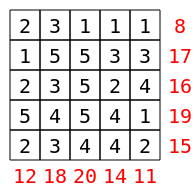CSES - Datatähti Open 2017 - Grid
• Time limit: 1.00 s
• Memory limit: 512 MB
Your task is to construct an $n \times n$ grid where each square contains an integer between $1 \ldots n$. There are two requirements:
1. Each integer $1 \ldots n$ appears exactly $n$ times in the grid.
2. The rows and columns should form $2n$ distinct sums.
For example, one possible $5 \times 5$ grid is as follows:Each number $1 \ldots 5$ appears $5$ times in the grid, and the sums of the rows and columns are $[8,11,12,14,15,16,17,18,19,20]$.

Input

The only input line contains an integer $n$.

Output

You should print any grid that fulfils the requirements. If there is no such grid, you should only print "QAQ".

Example 1

Input:
5

Output:
2 3 1 1 1 1 5 5 3 3 2 3 5 2 4 5 4 5 4 1 2 3 4 4 2

Example 2

Input:
2

Output:
QAQ

• $2 \le n \le 10$
• $2 \le n \le 100$
• $2 \le n \le 1000$